Warning: Illegal string offset 'html' in /home/hsn/public_html/forum/cache/skin_cache/cacheid_1/skin_topic.php on line 909

Homework Question - HSN forum# Homework Question

2 replies to this topic

### #1General_Fondue

• Location:Greenock
• Gender:Male

Posted 16 April 2008 - 12:40 PM

I am having some a lot of trouble with a question we were given for homework. It is:

QUOTE
The line y = -1 is a tangent to a circle which passes through (0,0) and (6,0). Find the Equation of the circle. (5 marks)

Can anyone help me with this problem?

### #2Gibbs

• Gender:Not Telling

Posted 16 April 2008 - 01:00 PM

I am having some a lot of trouble with a question we were given for homework. It is:

QUOTE
The line y = -1 is a tangent to a circle which passes through (0,0) and (6,0). Find the Equation of the circle. (5 marks)

Can anyone help me with this problem?

The point of Intersection with the line and the two co-ordinates must be (3,-1). this is because if u draw a graph of the circle you will see that these two points form an arc. halfway between these the x point would be 3 since its y=-1 the y point must be -1. Im not sure about the next but maybe it works lol.

Since the radius is perpendicular to any tanjent the the gradient of the raidus must also be zero.meaning that the x co ordinate is 3.

Im stuck on the next bit will keep think though

### #3Steve

Top of the Class

••• 435 posts
• Location:Edinburgh
• Gender:Male

Posted 16 April 2008 - 06:09 PM

There are probably a few ways you could do the next bit; here's one...

From the working in the last post, we know that the centre has coordinates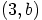where we don't know what b is.

In addition, we know that the radius of the circle is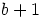(a diagram here would help!). So the equation is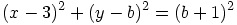We know that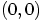lies on the circle so put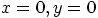into the equation to get: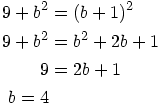So the equation is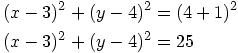Hope this helps.HSN contribute: Help the site grow!

Looking for a Maths tutor in West Lothian? Just PM me!

#### 1 user(s) are reading this topic

0 members, 1 guests, 0 anonymous users